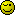# Get before comma in array?

I’m trying to figure this out…

I have a string like this:

``````\$string = '1,john,43,2,peter,51,3,jeff,50,5,nick,46';
``````

The string is like this: placement,name,age

So to get this out in an array I do this:

``````\$array = explode(",",\$string);
\$h = '';
\$k = 0;

foreach(\$array as \$value){
\$h .= (\$k%3 == 2)?\$value.';':\$value.',';
\$k++;
}

\$newarray = explode(";",substr(\$h,0,-1));
``````

Now I have an array like this

``````Array
(
 => 1,john,43
 => 2,peter,51
 => 3,jeff,50
 => 5,nick,46
)
``````

Now what I want now is to be able to get the placement out of the array and find the missing placement (4) and make that into an variable… Just dont know where to start?

Could you check that each array starts with the array item number + 1?

i.e. if “\$newarray == 4” then it is correct however if “\$newarray !== 4” then it is missing?

``````

for(\$i = 0,\$i <= count(\$newarray),\$i++){
\$missing = (substr(\$newarray[\$i], 0, 1) == (\$i + 1)) ? 'Value exists':'Value dosnt exist';
}

``````

I am by no means a PHP expert and I will be surprised if this even works (cant test it right now), but it might spark an idea?

Actually… It kind a works… When doing the script above I get this:
Value existsValue existsValue existsValue dosnt existValue dosnt exist

Now, how do I extract the number that is missing so I only get that and as a number e.g. number 4 in this case?

Oh awesome.

To get the number back:

``````
for(\$i = 0,\$i <= count(\$newarray),\$i++){
\$missing = (substr(\$newarray[\$i], 0, 1) == (\$i + 1)) ? 'Value for \$newarray[' . \$i . '] exists':'Value for \$newarray[' . \$i . '] dosnt exist';
}

``````

And then you need to run some if statement or several to incriment \$i because the numbers are out of sync, instead of the numbers being different by one they are now differrent by two and if another value fails they will become different by three etc etc I will be back later, ill try to think of a the next bit.

Now, mayby we are allmost there but not yet…

When doing this:

``````for (\$i = 0; \$i <= count(\$newarray); \$i++) {
\$missing = (substr(\$newarray[\$i], 0, 1) == (\$i + 1)) ? 'Value for '.substr(\$newarray[\$i], 0, 1).' exists<br>':'Value for '.substr(\$newarray[\$i], 0, 1).' dosnt exist<br>';
echo \$missing;
}
``````

I get this:

``````Value for 1 exists
Value for 2 exists
Value for 3 exists
Value for 5 dosnt exist
Value for dosnt exist
``````

It jumps number 4 and actually… Thats the only number I want? Where do I/we go wrong?

Well… Getting close…
I have done this:

``````\$string = '1,john,43,2,peter,51,3,jeff,50,5,nick,46,7,Rick,46';

\$array = explode(",",\$string);
\$h = '';
\$k = 0;

foreach(\$array as \$value){
\$h .= (\$k%3 == 2)?\$value.';':\$value.',';
\$k++;
}

\$newarray = explode(";",substr(\$h,0,-1));

for (\$i = 0; \$i <= count(\$newarray); \$i++) {
\$arr1[] = substr(\$newarray[\$i], 0, 1);
}

\$arr2 = range(1,max(\$arr1));

\$missing = array_diff(\$arr2,\$arr1);

echo '<pre>';
print_r(\$missing);
echo '</pre>';
``````

This gives me the 2 missing numbers as I wanted… But I only want to get the first missing number into an phh variable \$firstmissing…

Hop do I do so?

And the winner is…

``````\$first = reset(\$missing);
``````

Thanks for all the helpIf you don’t care about the “inbetween array” * you can also do this:

``````
\$string = '1,john,43,2,peter,51,3,jeff,50,5,nick,46,7,Rick,46';
\$arr = explode(',', \$string);
\$count = count(\$arr);
\$current = \$arr;

for (\$i=3; \$i<\$count; \$i+=3) {
\$expected = \$current+1;
if (\$arr[\$i] != \$expected) {
echo \$expected, ' is missing!';
}
\$current = \$arr[\$i];
}

``````

*) I mean this one

``````
Array
(
 => 1,john,43
 => 2,peter,51
 => 3,jeff,50
 => 5,nick,46
)

``````

haha That seemed so hard and yet you can just think of that solution! Looks good.